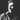# Partial function application and currying in Ruby

March 08, 2016

Currying and partial function application are common concepts of the functional programming. They look similar, but have differences in realization and using. Ruby allows you to easily operate with both of them.

## Partial function application

First we need to know what is really application is: it’s the process of applying function to all of it’s arguments to return value.
Partial function application is the process of applying function to some of it’s arguments. This process creates a new function, based on the parent function, but with lower arity (with fewer arguments). So, if we have an abstract functionwith three arguments, then we can create a partial functionwith two arguments that both of this functions return the same result.

For example, we have a simple multiply function that multiplies two arguments:

``````  multiply = -> (x, y) { x * y }
multiply.(2, 2) # 4``````

But what if we want just double numbers ? Should we pass the 2 argument each time? Not really. We can use partial function application to create a new double function that takes just one argument:

``````  double = -> (x) { multiply.(2, x) }
double.arity # 1
double.(2) # 4
double.(1984) # 3968``````

Ruby has Proc#curry method that allows you to use partial function application even more simpler:

``````  double = multiply.curry.(2)
double.(2) # 4
double.(300) # 600``````

## Currying

Currying is similar to partial function application concept.

Currying is the process of translating the evaluating of function with many arguments into evaluating a sequence of functions, each with exactly one parameter. So, if we have a function with two arguments:then we can translate it withtransformation to return a new function with one parameter:.

For example, look back at previous multiply function. What if we want to multiply more than two arguments?

``  multiply.(2, 2, 2) # ArgumentError: wrong number of arguments (given 3, expected 2)``

To prevent this, we can change multiply function and use Proc#curry method:

``````  multiply = -> (head, *tail) { head * tail.inject(1, &:*) }
multiply.curry.(2, 2, 2) # 8
multiply.curry.(2, 3, 7) # 42``````

If we want to restrict arguments count, we can use arity optional argument in Proc#curry function:

``````  multiply.curry(3) # 6
multiply.curry(3) # 0 because of the last argument is nil``````

You can also use curry method on plain methods, not only procs with Ruby 2.2:

``````  def sum(*args)
args.inject(:+)
end

plus_two = method(:sum).curry(2).(2)
plus_two.(3) # 5``````

## Conclusion

Partial function application and currying are both great features of functional programming that allows you to write clean, simple and flexible functions based on anothers. You can use Ruby’s #curry method with procs or methods to write eloquent and powerful code.

Tags:Aleksei Kuznetsov

Functional programming, software architecture, DevOps

Salam